# WBJEE 2016 Maths Paper with Solutions

WBJEE 2016 Mathematics solved paper is available here. All exam questions are solved by expert teachers. Solving these questions will help the candidates to get an idea about the question paper pattern, the number of questions asked in Mathematics, the simplest method to solve the questions, etc. Students are advised to download the WBJEE 2016 Mathematics paper to practise and score well in the exam.

### WBJEE 2016 - Maths

Question 1: Let A and B be two events such that

$$P(A\cap B)=\frac{1}{6},P(A\cup B)=\frac{31}{45}$$
and
$$P(\bar{B})=\frac{7}{10}$$
then

1. a. A and B are independent
2. b. A and B are mutually exclusive
3. c.
$$P(\frac{A}{B})< \frac{1}{6}$$
4. d.
$$P(\frac{B}{A})< \frac{1}{6}$$

Solution:

$$P(A\cap B)=\frac{1}{6},P(A\cup B)=\frac{31}{45}$$
and
$$P(\bar{B})=\frac{7}{10}$$

$$P(B)=1-\frac{7}{10}=\frac{3}{10}$$

$$\Rightarrow P(A\cup B)=P(A)+P(B)-P(A\cap B)$$

$$\Rightarrow \frac{31}{45} =P(A)+\frac{3}{10}-\frac{1}{6}\Rightarrow P(A)=\frac{5}{9}$$

Therefore,

$$P(A)\times P(B)=\frac{5}{9}\times \frac{3}{10}=\frac{1}{6}=P(A\cap B)$$

$$\Rightarrow$$
A, B are independent events

Question 2: The value of

$$cos15^{0}cos7\frac{1^{0}}{2}sin7\frac{1^{0}}{2}$$
is

1. a. ½
2. b. 1/8
3. c. ¼
4. d. 1/16

Solution:

$$cos15^{0}cos7\frac{1^{0}}{2}sin7\frac{1^{0}}{2}$$

$$\frac{2cos15^{0}cos7\frac{1^{0}}{2}sin7\frac{1^{0}}{2} }{2}$$

$$\frac{sin15^{0}cos15^{0}}{2}\times \frac{2}{2}$$

$$\Rightarrow \frac{sin30^{0}}{4}=\frac{1}{8}$$

Question 3:The smallest positive root of the equation tan x – x = 0 lies in

1. a. (0, π/2)
2. b.( π/2, π)
3. c.( π, 3π/2)
4. d. (3π/2, 2π)

Solution:

tan x = x

y = tan x ……….(1)

y = x ………….. (2)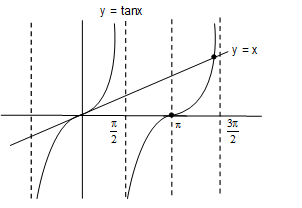It is clearly visible that solution lies in ( π, 3π/2)

Question 4: If in a triangle ABC, AD, BE and CF are the altitudes and R is the circumradius, then the radius of the circumcircle of ΔDEF is

1. a. R/2
2. b. 2R/3
3. c.(1/3)R
4. d. None of these

Solution:

Here, DEF is a pedal triangle of ΔABC

We know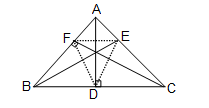EF = a cosA = R sin2A (side of pedal Δ)

$$\angle FDE = 180^{0}-2A$$

Let, circumradius of ΔDEF be R’.

Now by sine rule in ΔDEF

$$2R^{'}=\frac{EF}{sin\angle FDE}=\frac{Rsin 2A}{sin (180^{0}-2A)}\Rightarrow R' =\frac{R}{2}$$

Question 5: The points (–a, –b), (a, b), (0, 0) and (a2, ab), a ≠0, b ≠ 0 always lie on this line. Hence, collinear

1. a. collinear
2. b. vertices of a parallelogram
3. c. vertices of a rectangle
4. d. lie on a circle

Solution:

$$\Delta =\frac{1}{2}\begin{vmatrix} -a & -b\\ a& b\\ 0 & 0\\ a^{2} & ab\\ -a& -b \end{vmatrix}$$

$$\Delta =\frac{1}{2}(-ab+ab+0-0+0-0-a^{2}b+a^{2}b)$$

$$\Delta =0$$

Hence, points are collinear

Question 6: The line AB cuts off equal intercepts 2a from the axes. From any point P on the line AB perpendiculars PR and PS are drawn on the axes. Locus of mid-point of RS is

1. a. x-y = a/2
2. b. x + y = a
3. c. x2 + y2 = 4a2
4. d. x2 – y2 = 2a2

Solution:

Equation of line which cuts equal intercepts from axes is x + y = 2a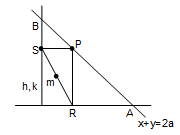Let, co-ordinates of the midpoint be m (h, k).

So, R and S are (2h, 0) and (0, 2k)

Therefore, p must be (2h, 2k)

Since, P lies on AB

Therefore, 2h + 2k = 2a

⇒x + y = a

Question 7: x + 8y – 22 = 0, 5x + 2y – 34 = 0, 2x – 3y + 13 = 0 are the three sides of a triangle. The area of the triangle is

1. a. 36 square unit
2. b. 19 square unit
3. c. 42 square unit
4. d. 72 square unit

Solution:

L1: x + 8y = 22

L2: 5x + 2y = 34

L3: 2x – 3y = –13

On solving L1, L2 & L3 we get

A = (–2, 3), B = (6, 2) and C = (4, 7)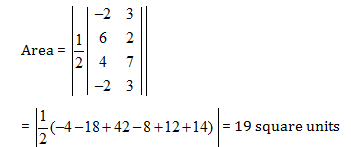Question 8: The line through the points (a, b) and (–a, –b) passes through the point

1. a. (1, 1)
2. b. (3a, –2b)
3. c. (a2, ab)
4. d. (a, b)

Solution:

Equation of line passes through points (a, b) & (–a, –b) is

$$\Rightarrow (y-b)=\frac{-2b}{-2a}(x-a)$$

⇒ay – ab = bx – ab

⇒ay = bx

Now check options, (C) & (D) are correct

Question 9:The locus of the point of intersection of the straight lines

$$\frac{x}{a}+\frac{y}{b}=K$$
and
$$\frac{x}{a}-\frac{y}{b}=\frac{1}{k}$$
where k is a non-zero real variable, is given by

1. a. a straight line
2. b. an ellipse
3. c. a parabola
4. d. a hyperbola

Solution:

$$L_{1}=\frac{x}{a}+\frac{y}{b}=k$$

$$L_{2}=\frac{x}{a}-\frac{y}{b}=\frac{1}{k}$$

Let point of intersection be

$$(\alpha ,\beta )$$

So,

$$\frac{\alpha }{a}+\frac{\beta }{b}=k \: and \: \frac{\alpha }{a}-\frac{\beta }{b}=\frac{1}{k}$$

$$\left [ \frac{\alpha }{a}+\frac{\beta }{b} \right ]\left [ \frac{\alpha }{a}-\frac{\beta }{b} \right ]=1$$

$$\left [ \frac{\alpha^{2} }{a^{2}}-\frac{\beta^{2} }{b^{2}} \right ]=1$$

$$Locus:\left [ \frac{x^{2} }{a^{2}}-\frac{y^{2} }{b^{2}} \right ]=1$$

Which is a hyperbola

Question 10: The equation of a line parallel to the line 3x + 4y = 0 and touching the circle x2 + y2 = 9 in the first quadrant is

1. a. 3x + 4y = 15
2. b. 3x + 4y = 45
3. c. 3x + 4y = 9
4. d. 3x + 4y = 27

Solution:

Let, equation of line which is parallel to given line is

3x + 4y = k

This line is tangent to circle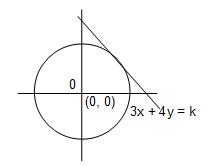⇒d = r

$$\left | \frac{0+0-k}{5} \right |=3$$

$$k=\pm 15$$

So, equation of tangent in first quadrant is 3x + 4y = 15

Question 11: A line passing through the point of intersection of x+y=4 and x–y=2 makes an angle

$$tan^{-1}(3/4)$$
with the x-axis. It intersects the parabola y2=4(x–3) at points (x1, y1) and (x2, y2) respectively. Then |x1–x2| is equal to

1. a. 16/9
2. b. 32/9
3. c. 40/9
4. d. 80/9

Solution:

L1: x + y = 4

L2: x – y = 2

line passing through the point of intersection is

⇒L = L1 + λL2 = 0

⇒ L = (x + y – 4) + λ (x – y – 2) =0

⇒L = x(1 + λ) + y (1 – λ) – 4 – 2 λ = 0

⇒ML = (given)

$$\Rightarrow \frac{1+\lambda }{-1+\lambda }=\frac{3}{4}$$

⇒λ= –7

Equation of line is L = –6x + 8y + 10 = 0

y = (3x-5)/4 (put in equation of parabola)

$$\Rightarrow \left ( \frac{3x-5}{4} \right )^{2}=4(x-3)\Rightarrow 9x^{2}-94x+217=0$$

$$\Rightarrow x_{1}+x_{2}=\frac{94}{9},x_{1}x_{2}=\frac{217}{9}$$

$$\Rightarrow \left | x_{1}-x_{2} \right |=\sqrt{(x_{1}+x_{2})^{2}-4x_{1}x_{2}}=\frac{32}{9}$$

Question 12: The equation of auxiliary circle of the 16x2+25y2+32x–100y=284 is

1. a. x2 + y2 + 2x – 4y – 20 = 0
2. b. x2 + y2 + 2x – 4y = 0
3. c. (x+1)2+(y–2)2 = 400
4. d. (x+1)2+(y–2)2=225

Solution:

⇒ 16x2 + 25y2 + 32x – 100y = 284

⇒16(x2 + 2x) + 25(y2 - 4y) = 284

⇒ 16 (x + 1)2 + 25(y – 2)2 = 284 + 16 +100

⇒ 16 (x + 1)2 + 25(y – 2)2 = 400

$$\frac{(x+1)^{2}}{25}+\frac{(y-2)^{2}}{16}=1$$

So, the auxiliary circle is (x + 1)2 + (y – 2)2 = 25

⇒ x2 + y2 + 2x – 4y – 20 = 0

Question 13: If PQ is a double ordinate of the hyperbola

$$\frac{x^{2}}{a^{2}}-\frac{y^{2}}{b^{2}}=1$$
such that ΔOPQ is equilateral, O being the centre. Then the eccentricity e satisfies

1. a.
$$1< e< \frac{2}{\sqrt{3}}$$
2. b.
$$e=\frac{2}{\sqrt{2}}$$
3. c.
$$e=\frac{\sqrt{3}}{2}$$
4. d.
$$e> \frac{2}{\sqrt{3}}$$

Solution:

Because, OPQ is equilateral

(OP)2 = (PQ)2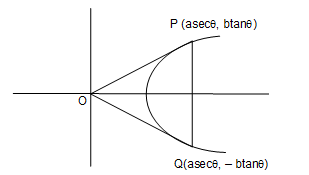⇒ a2sec2 θ + b2 tan2θ = (2b tanθ)2

⇒a2sec2 θ = 3b2 tan2 θ

⇒ sin2θ = (a2/3b2)

⇒ Now, sin2 θ < 1

$$\Rightarrow \frac{a^{2}}{3b^{2}}< 1$$

$$\Rightarrow \frac{b^{2}}{a^{2}}> \frac{1}{3}$$

On adding 1 both sides

$$\Rightarrow 1+\frac{b^{2}}{a^{2}}>1+ \frac{1}{3}$$

$$\Rightarrow e^{2}> \frac{4}{3}$$

On taking root both sides

$$\Rightarrow e> \frac{2}{\sqrt{3}}$$

Question 14: If the vertex of the conic y2 – 4y = 4x – 4a always lies between the straight lines; x+y=3 and 2x+2y–1=0 then

1. a. 2<a<4
2. b. (-1/2) <a<2
3. c. 0<a<2
4. d.(-1/2) < a<(3/2)

Solution:

⇒y2 – 4y = 4x – 4a

⇒ y2 – 4y + 4 = 4x – 4a + 4

⇒ (y – 2)2 = 4(x–(a–1))

Therefore, Vertex: (a–1, 2)

Vertex lies in between lines

L1 × L2 < 0

⇒ (a – 1 + 2 – 3) × (2(a–1) + 4 – 1) < 0

⇒ (a – 2) (2a + 1) < 0

$$\Rightarrow a\epsilon \left [ \frac{-1}{2},2 \right ]$$

Question 15: A straight line joining the points (1,1,1) and (0,0,0) intersects the plane 2x+2y+z=10 at

1. a. (1, 2, 5)
2. b. (2, 2, 2)
3. c. (2, 1, 5)
4. d. (1, 1, 6)

Solution:

Equation of line joining points (1, 1, 1) & (0, 0, 0) is

$$\Rightarrow L =\frac{x-0}{1-0}=\frac{y-0}{1-0}=\frac{z-0}{1-0}$$

i.e. L : x = y = z = k (let) ……..(1)

Any point on L is p(k, k, k)

Since line & plane intersect at a point so, P lies on 2x + 2y + z = 10

⇒2k + 2k + k = 10

⇒ k = 2

So, point is (2, 2, 2)

Question 16: Angle between the planes x+y+2z=6 and 2x–y+z=9 is

1. a. π/4
2. b. π/6
3. c. π/3
4. d. π/2

Solution:

Angle between planes = cos θ =

$$\frac{a_{1}b_{1}+a_{2}b_{2}+a_{3}b_{3}}{\sqrt{a_{1}^{2}+a_{2}^{2}+a_{3}^{2}}.\sqrt{b_{1}^{2}+b_{2}^{2}+b_{3}^{2}}}$$

⇒ cos θ =

$$\frac{1\times 2+1\times (-1)+2\times 1}{\sqrt{1^{2}+1^{2}+2^{2}}.\sqrt{2^{2}+(-1)^{2}+1^{2}}}$$

⇒cos θ = 3/6

⇒ cos θ =1/2

⇒θ = π/3

Question 17: If y =(1+x)(1+x2)(1+x4)........(1+x2n) then the value of at x = 0 is

1. a. 0
2. b. –1
3. c. 1
4. d. 2

Solution:

y = (1 + x) (1 + x2) (1+ x4) ………..(1 + x2n)

Taking log both sides

log y = log(1 + x) + log (1 + x2) + ……… + log(1 + x2n)

On differentiating both sides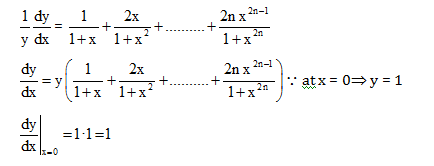Question 18: If f(x) is an odd differentiable function defined on (–∞,∞) such that f' (3) = 2, then f' (–3) equal to

1. a. 0
2. b. 1
3. c. 2
4. d.4

Solution:

f(x) is odd differentiable

f(–x) = –f(x)

On differentiating both sides

⇒ –f ‘(–x) = – f ‘(x)

⇒f ‘(x) = f ‘(–x) ……..(1)

⇒put x = 3 in eq. (1)

⇒ f ‘(3) = f’(–3)

⇒ f ‘(–3) = 2

since f ‘(3) = 2 (given)

Question 19:

$$\lim_{x\rightarrow 1}\left ( \frac{1+x}{2+x} \right )^{\frac{1-\sqrt{x}}{1-x}}$$

1. a. is 1
2. b. does not exist
3. c. is
$$\sqrt{\frac{2}{3}}$$
4. d. is/n 2

Solution: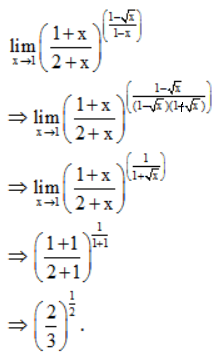Question 20: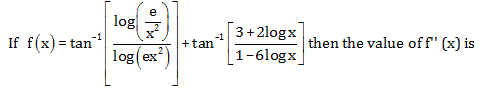1. a. x2
2. b. x
3. c. 1
4. d. 0

Solution: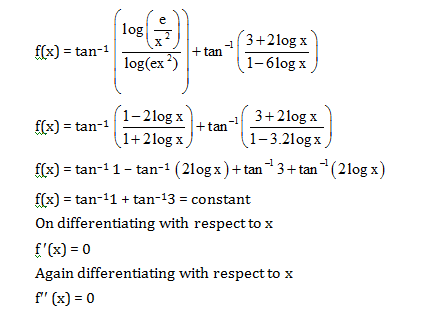Question 21:

$$\int \frac{log\sqrt{x}}{3x}dx$$
is equal to

1. a.
$$\frac{1}{3}\left ( log\sqrt{x} \right )^{2}+c$$
2. b.
$$\frac{2}{3}\left ( log\sqrt{x} \right )^{2}+c$$
3. c.
$$\frac{2}{3}\left ( logx \right )^{2}+c$$
4. d.
$$\frac{1}{3}\left ( logx \right )^{2}+c$$

Solution:

$$I=\int \frac{log\sqrt{x}}{3x}dx$$

Let

$$log\sqrt{x}=t$$

$$\Rightarrow \frac{1}{\sqrt{x}}.\frac{1}{2\sqrt{x}}dx=dt\Rightarrow \frac{dx}{x}=2dt$$

$$\Rightarrow I=\int \frac{t}{3}2dt$$

$$\Rightarrow I=\frac{2}{3}\frac{t^{2}}{2}+c\Rightarrow \frac{1}{3}(log\sqrt{x})^{2}+c$$

Question 22:

$$\int 2^{x}(f'(x)+f(x)log 2)$$
is equal to

1. a. 2xf’(x) + c
2. b. 2xlog2+c
3. c. 2xf(x) + c
4. d. 2x+c

Solution:

$$I=\int 2^{x}(f'(x)+f(x)log2)$$

Let g(x)=2xf(x) ⇒g’(x)=2xf’(x)+f(x)2xln2

$$I=\int g'(x)dx$$

$$I=g(x)+C$$

I= 2xf(x)+c

Question 23:

$$\int_{0}^{1}log(\frac{1}{x}-1)dx$$
=

1. a. 1
2. b. 0
3. c. 2
4. d. None of these

Solution:

$$I=\int_{0}^{1}log(\frac{1}{x}-1)dx$$

$$I=\int_{0}^{1}log(\frac{1-x}{x})dx$$

On applying king property

$$I=\int_{0}^{1}log(\frac{x}{1-x})dx$$

$$=-\int_{0}^{1}log(\frac{1-x}{x})dx=-I$$

⇒I =-I

⇒2I=0

⇒ I =0

Question 24: The value of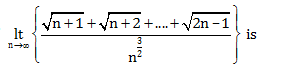1. a.
$$\frac{2}{3}(2\sqrt{2}-1)$$
2. b.
$$\frac{2}{3}(\sqrt{2}-1)$$
3. c.
$$\frac{2}{3}(2\sqrt{2}+1)$$
4. d.
$$\frac{2}{3}(2\sqrt{2}+1)$$

Solution: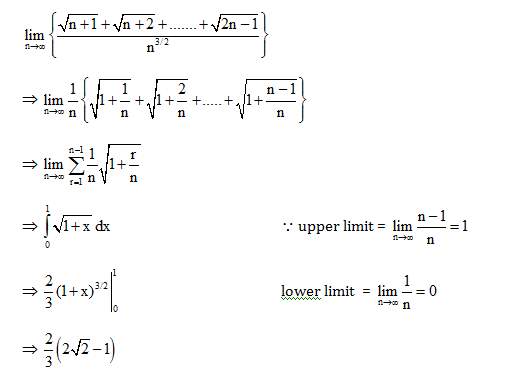Question 25: If the solution of the differential equation be

$$x\frac{dy}{dx}+y=xe^{x}$$
be ,
$$xy=e^{x}\phi (x)+c$$
then
$$\phi (x)$$
is equal to

1. a. x+1
2. b. x–1
3. c. 1–x
4. d. x

Solution:

$$x\frac{dy}{dx}+y=xe^{x}$$

$$\frac{dy}{dx}+\frac{y}{x}=e^{x}$$
----- linear differential equation

I.F=

$$e^{\int \frac{1}{x}dx}=e^{lnx}=x$$

Solution of L.D.E

⇒y. x =

$$\int e^x.x.dx$$

⇒ y . x = xex

$$\int e^xdx$$

⇒y.x = xex – ex + c

⇒ xy = ex(x – 1) + c

On comparing with given relation

$$xy=e^{x}\phi (x)+c$$

φ (x) = (x–1)

Question 26: The order of the differential equation of all parabolas whose axis of symmetry along x-axis is

1. a. 2
2. b. 3
3. c. 1
4. d. None of these

Solution:

Let, equation of parabola y2 = 4a (x – b) ……..(1)

On differentiating with respect to x

⇒2yy’ = 4a

Again differentiating with respect to x

⇒ 2yy’’ + 2(y’)2 = 0

Order of differential equation = 2

Question 27: The line y = x + λ is tangent to the ellipse 2x2 + 3y2 = 1. Then λ is

1. a. –2
2. b. 1
3. c.
$$\sqrt{\frac{5}{6}}$$
4. d.
$$\sqrt{\frac{2}{3}}$$

Solution:

$$E:\frac{x^{2}}{3}+\frac{y^{2}}{2}=\frac{1}{6}$$
---------(1)

L: y = x + λ …………(2)

Line is tangent to ellipse

⇒ 2x2 + 3 (x + λ)2 = 1

⇒ 2x2 + 3 (x2 + 2x λ + λ2) =1

⇒ 5x2 + 6xλ + 3λ2 – 1 = 0

⇒ D = 0

⇒ 36λ2 – 20 (3 λ2–1) = 0

⇒ – 24λ2 + 20 = 0 ⇒ 6 λ2 – 5 = 0

$$\lambda =\pm \sqrt{\frac{5}{6}}$$

Question 28: The area enclosed by

$$y=\sqrt{5-x^{2}}$$
and
$$y=\left | x-1 \right |$$

1. a.
$$\left [ \frac{5\pi }{4}-2 \right ]$$
sq.units
2. b.
$$\frac{5\pi -2}{2}$$
sq.units
3. c.
$$\left [ \frac{5\pi }{4}-\frac{1}{2} \right ]$$
sq.units
4. d.
$$\left [ \frac{\pi }{2}-5 \right ]$$
sq.units

Solution:

$$y=\sqrt{5-x^{2}}$$
…… (1)

y = |x – 1| …… (2)

From equation (1) & (2)

⇒5 – x2 = (x– 1)2

⇒5 – x2 = x2 –2x + 1

⇒ 2x2 – 2x – 4 = 0

⇒x2 – x – 2 = 0 ⇒ x = –1, 2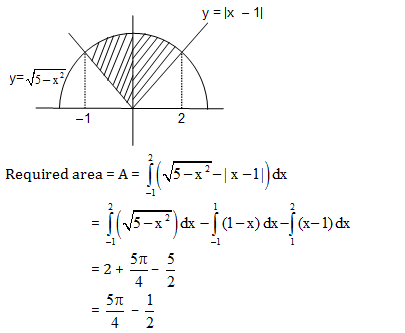Question 29: Let S be the set of points whose abscissas and ordinates are natural numbers. Let P ∈S such that the sum of the distance of P from (8,0) and (0,12) is minimum among all elements in S. Then the number of such points P in S is

1. a. 1
2. b. 3
3. c. 5
4. d. 11

Solution:Question 30: Time period T of a simple pendulum of length l is given by

$$T=2\pi \sqrt{\frac{l}{g}}$$
. If the length is increased by 2%, then an approximate change in the time period is

1. a. 2%
2. b. 1%
3. c. ½ %
4. d. None of these

Solution:

we have

$$T=2\pi \sqrt{\frac{l}{g}}$$

Taking log both sides

log T = log 2 π +(1/2) (log l – log g)

Differentiating both sides we get

$$\Rightarrow \frac{1}{T}dT=\frac{1}{2}\frac{1}{l}dl$$

$$\Rightarrow \frac{1}{T}dT\times 100=\frac{1}{2}\left [ \frac{dl}{l}\times 100 \right ]=\frac{1}{2}\times 2=1%$$

Question 31: The cosine of the angle between any two diagonals of a cube is

1. a. 1/3
2. b. 1/2
3. c. 2/3
4. d.
$$\frac{1}{\sqrt{3}}$$

Solution:

Direction cosine of diagonal OD =

$$\left [ \frac{1}{\sqrt{3},}\frac{1}{\sqrt{3}},\frac{1}{\sqrt{3}} \right ]$$

Direction cosine of diagonal FB =

$$\left [ \frac{1}{\sqrt{3},}\frac{1}{\sqrt{3}},\frac{-1}{\sqrt{3}} \right ]$$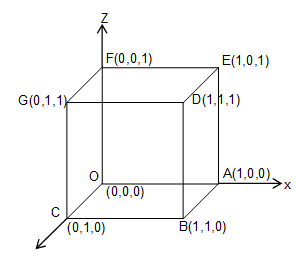$$Cos\theta =\left [ \frac{1}{\sqrt{3}}.\frac{1}{\sqrt{3}}+\frac{1}{\sqrt{3}}.\frac{1}{\sqrt{3}}+\frac{-1}{\sqrt{3}}.\frac{-1}{\sqrt{3}} \right ]=\frac{1}{3}$$

Question 32: If x is a positive real number different from 1 such that logax, logbx, logcx are in A.P., then

1. a.
$$b=\frac{a+c}{2}$$
2. b.
$$b=\sqrt{ac}$$
3. c.
$$c^{2}=(ac)^{log_{a}b}$$
4. d. None of (A), (B), (C) are correct

Solution:

logax, logbx, logcx

$$\rightarrow$$
A.P

Then,

⇒ 2logbx=logax+logcx

$$\frac{2}{log_{x}b}=\frac{1}{log_{x}a}+\frac{1}{log_{x}c}$$

⇒ 2logxalogxc=logxac.logxb

⇒ logxc2 =logxac.logxb

⇒ c2 = (ac)logab

Question 33: If a, x are real numbers and |a| < 1, |x| < 1, then 1 + (1+a)x + (1+a+a2)x2 + .... ∞is equal to

1. a.
$$\frac{1}{(1-a)(1-ax)}$$
2. b.
$$\frac{1}{(1-a)(1-x)}$$
3. c.
$$\frac{1}{(1-x)(1-ax)}$$
4. d.
$$\frac{1}{(1-a)(1+ax)}$$

Solution:

1 + (1+a)x + (1+a+a2)x2 + ....

Multiply & divide by (1 – a) we get

= 1/(1-a)[(1-a)+(1-a2)x + (1-a3)x2+ .... ∞]

$$=\frac{1}{1-a}\left [ \frac{1}{1-x}-\frac{1}{1-ax} \right ]$$

$$=\frac{1}{1-a}\left [ \frac{1-ax-a+ax}{(1-x)(1-ax)}\right ]$$

=

$$\frac{1}{(1-x)(1-ax)}$$

Question 34: If log0.3 (x–1) < log0.09 (x–1), then x lies in the interval

1. a. (2, ∞)
2. b. (1, 2)
3. c. (–2, –1)
4. d. None of these

Solution:

Log3(x-1) <log9(x-1) since x-1>0⇒x>1 ---------(1)

⇒ log3(x-1) < log(3)2(x-1)

⇒ 2log3(x-1) < log(3)(x-1)

⇒(x-1)2 >(x-1)

⇒x2-3x+2>0

$$x\epsilon (-\infty,1)\cup (2,\infty )$$
--------(2)

From (1) & (2)

$$x\epsilon (2,\infty )$$

Question 35: The value of

$$\sum_{n=1}^{13}(i^{n}+i^{n+1}),i=\sqrt{-1}$$
is

1. a. i
2. b. i–1
3. c. 1
4. d. 0

Solution:

$$\sum_{n=1}^{13}(i^{n}+i^{n+1})$$

Since we know,

i + i2 + i3 + i4 = 0

$$\sum_{n=1}^{13}i^{n}+\sum_{n=1}^{13}i^{n+1}$$

⇒ i13+i14

⇒ i-1

Question 36: If z1, z2, z3 are imaginary numbers such that |z1| = |z2| = |z3|=

$$|\frac{1}{z_1}+\frac{1}{z_2}+\frac{1}{z_3}|$$
= 1 then |z1 +z2+z3| is

1. a. Equal to 1
2. b. Less than 1
3. c. Grater than 1
4. d. Equal to 3

Solution:

$$\left | \frac{1}{z_{1}}+\frac{1}{z_{2}}+\frac{1}{z_{3}}\right |=1$$

Since

$$z\bar{z}=\left | z \right |^{2}=1\Rightarrow \bar{z}=\frac{1}{z}$$

$$\Rightarrow \left | \bar{z_{1}}+\bar{z_{2}}+\bar{z_{3}} \right |=1$$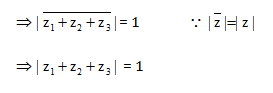Question 37: If p, q are the roots of the equation x2 + px + q = 0, then

1. a. p = 1, q = –2
2. b. p = 0, q = 1
3. c. p = –2, q = 0
4. d.p = –2, q = 1

Solution: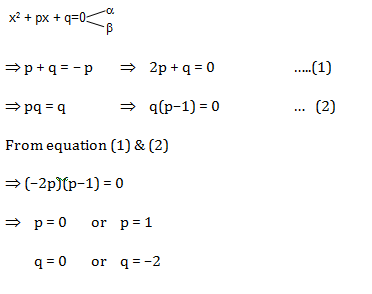Question 38: The number of values of k for which the equation x2 – 3x + k = 0 has two distinct roots lying in the interval (0, 1) are

1. a. Three
2. b. Two
3. c. Infinitely many
4. d. No values of k satisfies the requirement

Solution:

(1) D > 0

9 – 4 k > 0

k < (9/4) …..(1)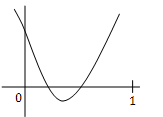(2) f(0) > 0

⇒ k > 0 …… (2)

(3) f(1) > 0

⇒1 – 3 + k > 0

⇒ k > 2 …..(3)

From (1), (2) & (3)

$$k\epsilon \left [ 2,\frac{9}{4} \right ]$$

Question 39: The number of ways in which the letters of the word ARRANGE can be permuted such that the R’s occur together is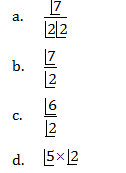Solution: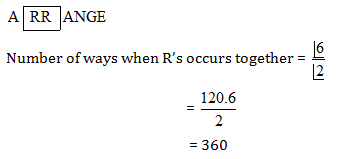Question 40: If

$$\frac{1}{^{5}C_{r}}+\frac{1}{^{6}C_{r}}=\frac{1}{^{4}C_{r}}$$
, then the value of r equals to

1. a. 4
2. b. 2
3. c. 5
4. d. 3

Solution:

$$\frac{1}{^{5}C_{r}}+\frac{1}{^{6}C_{r}}=\frac{1}{^{4}C_{r}}$$

$$\Rightarrow \frac{r!(5-r)!}{5!}+\frac{r!(6-r)!}{6!}=\frac{r!(4-r)!}{4!}$$

⇒ 6(5 – r) + (6 – r) (5 – r) = 6 × 5

⇒30 – 6r + r2 – 11 r + 30 = 30

⇒ r2 – 17r + 30 = 0

⇒r = 2, 15 (not possible)

⇒r = 2

Question 41: For +ve integer n, n3 + 2n is always divisible

1. a. 3
2. b. 7
3. c. 5
4. d. 6

Solution:

n3 + 2n, where n is positive integer i.e. n = 1, 2, 3

When

n = 1, 13 + 2.1 = 3 = 3 × 1

n = 2, 23 + 2.2 = 12 = 3 × 4

n = 3, 33 + 2×3 = 33 = 3 × 11

n = 4, 43 + 2×4 = 72 = 3 × 24

n = 5, 53 + 2×5 = 135 = 3 × 45

Hence, n3 + 3n is divisible by 3

Option (a) is correct

Question 42: In the expansion of (x – 1) (x – 2) .... (x – 18), the coefficient of x17 is

1. a. 684
2. b. –171
3. c. 171
4. d. –342

Solution:

(x –1) (x–2) (x–3) ….. (x–18)

(x –1) (x–2) = x2–3x + 2, coefficient of x = –3 = –(1+2)

(x –1) (x–2) (x–3) = (x2–3x + 2) (x – 3)

= x3 – 6x2 + 11x –6, coefficient of x2 = – 6 = –(1 + 2 + 3)

As, similarly,

Coefficient of x17 = –(1+2+3+……+18)

= –(18(18+1)/2)

= – 9 × 19 = –171

Hence option (b) is correct

Question 43:

$$1+^{n}C_{1}cos\theta +^{n}C_{2}cos2\theta +-------+^{n}C_{n}cosn\theta$$
equals

1. a.
$$\left [ 2cos\frac{\theta }{2} \right ]^{n}cos\frac{n\theta }{2}$$
2. b.
$$2cos^{2}\frac{n\theta }{2}$$
3. c.
$$2cos^{2n}\frac{\theta }{2}$$
4. d.
$$\left [ 2cos^{2}\frac{\theta }{2} \right ]^{n}$$

Solution: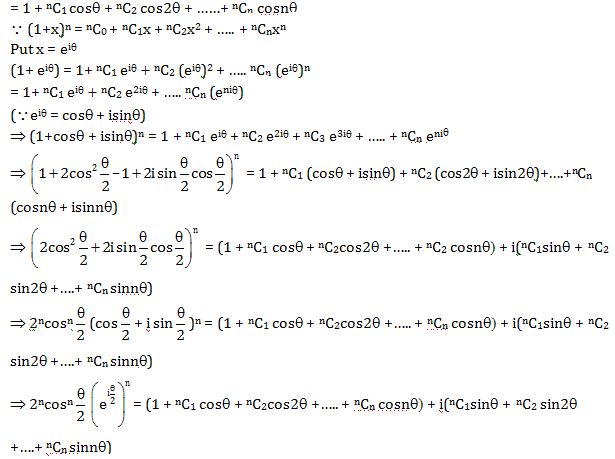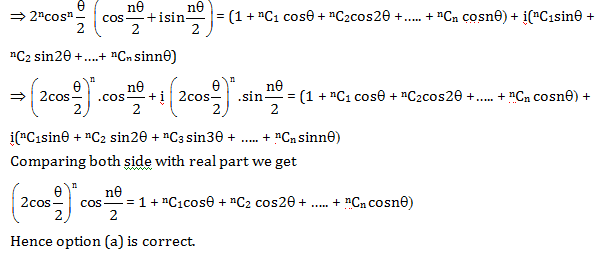Question 44. If x, y and z be greater than 1, then the value of

$$\begin{vmatrix} 1 & log_{x}y &log_{x}z \\ log_{y}x & 1 &log_{y}z \\ log_{z}x &log_{z}y & 1 \end{vmatrix}$$
is

1. a. log x. logy. log z
2. b. log x + logy + log z
3. c. 0
4. d. 1 – {(log x).(logy).(logz)}

Solution: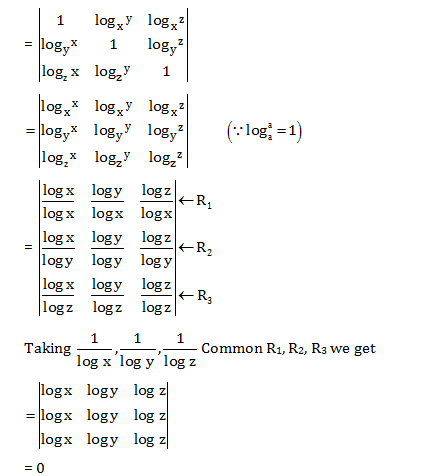Question 45: Let A is a 3 × 3 matrix and B is its adjoint matrix. If |B| = 64, then |A| =

1. a. ±2
2. b. ±4
3. c. ±8
4. d. ±12

Solution:

B is adjoint matrix of A

⇒ B = adj(A)

⇒ |B| = |adj(A)|

⇒ 64 = |adj(A)| (since|B| = 64)

⇒|A|(n–1) = 64 (since n = 3)

⇒|A|(3–1) = 64

⇒|A|2 = 82

⇒|A| = ±8

Hence option (c) is correct.

Question 46: Let Q =

$$\begin{bmatrix} cos\frac{\pi }{4} & sin\frac{\pi }{4}\\ sin\frac{\pi }{4} & cos\frac{\pi }{4} \end{bmatrix}$$
and x=
$$\begin{bmatrix} \frac{1}{\sqrt{2}}\\ \frac{1}{\sqrt{2}} \end{bmatrix}$$
then Q3x is equal to

1. a.
$$\begin{bmatrix} 0\\ 1 \end{bmatrix}$$
2. b.
$$\begin{bmatrix} \frac{1}{\sqrt{2}}\\ \frac{1}{\sqrt{2}} \end{bmatrix}$$
3. c.
$$\begin{bmatrix} -1\\ 0 \end{bmatrix}$$
4. d.
$$\begin{bmatrix} \frac{-1}{\sqrt{2}}\\ \frac{1}{\sqrt{2}} \end{bmatrix}$$

Solution: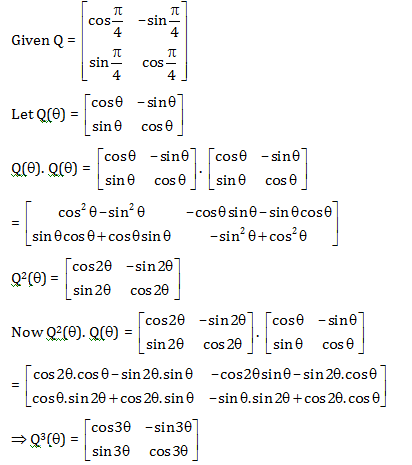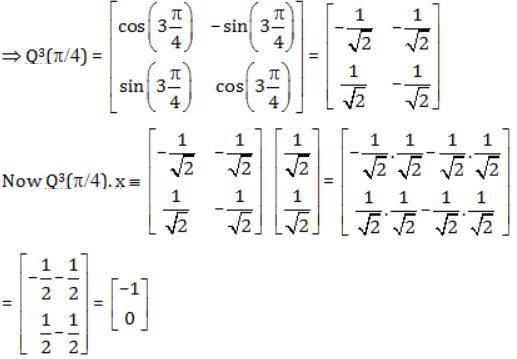Question 47: Let R be a relation defined on the set Z of all integers and xRy when x + 2y is divisible by 3. Then

1. a. R is not transitive
2. b. R is symmetric only
3. c. R is an equivalence relation
4. d. R is not an equivalence relation

Solution:

R = {x, y : x, y ∈ z, x + 2y is divisible by 3}

Reflexive: Let x, y ∈z

x = y

x + 2x = 3x

it is divisible by 3

(x, x) ∈R

So it is reflexive

Symmetric: If x R y ⇒ x + 2y is divisible by 3.

Now, y + 2x = 3x + 3y – (x + 2y) is divisible by 3.

⇒ y R x

i.e. it is symmetric.

Transitive: x R y ⇒x + 2y is divisible by 3.

y R z ⇒ y + 2z is divisible by 3.

⇒ x + 2y + y + 2z is divisible by 3.

⇒x + 3y + 2z is divisible by 3.

⇒x + 2z is divisible by 3.

⇒x R z

Hence transitive

Therefore, R is equivalence relation.

Question 48: If

$$A={5^{n}-4n-1:n\epsilon N}$$
and
$$B={16(n-1):n\epsilon N}$$
,then

1. a. A= B
2. b.
$$A\cap B=\phi$$
3. c.
$$A\subseteq B$$
4. d.
$$B\subseteq A$$

Solution:

$$A={5^{n}-4n-1:n\epsilon N}$$

When n = 1, 51 – 4 × 1–1 = 0

n = 2, 52 – 4 × 2–1 = 16

n = 3, 53 – 4 × 3–1 = 112

n = 4, 54 – 4 × 4–1 = 608

. . . . . . . . . . . . . . . . . . .

⇒ A = {0, 16, 112, 608}

While, B = {16(n–1), n ∈ N}

B = {0, 16, 32, 48……}

Hence it is clear that A ⊆ B

Hence option (c) is correct.

Question 49: If the function f: R

$$\rightarrow$$
R is defined by f(x) = (x2+1)35
$$\forall$$
x ∈R, then f is

1. a. one-one but not onto
2. b. onto but not one-one
3. c. neither one-one nor onto
4. d. both one-one and onto

Solution:

f(x) = (x2 + 1)35.

$$\forall$$
x∈ R

f(–x) = ((–x)2 +1)35

= (x2 +1)35 = f(x)

Hence f(x) is an even function

So its range ≠ R (f(x) > 0

$$\forall$$
x ∈ R)

And also it is not one-one and not onto.

Hence option (c) is correct.

Question 50: Standard Deviation of n observations a1, a2, a3 .....an is σ. Then the standard deviation of the observations λa1 , λa2 …… λan is

1. a. λσ
2. b. -λσ
3. c.
$$\left | \lambda \right |\sigma$$
4. d. λnσ

Solution:

Observations are a1, a2, a3 ……. an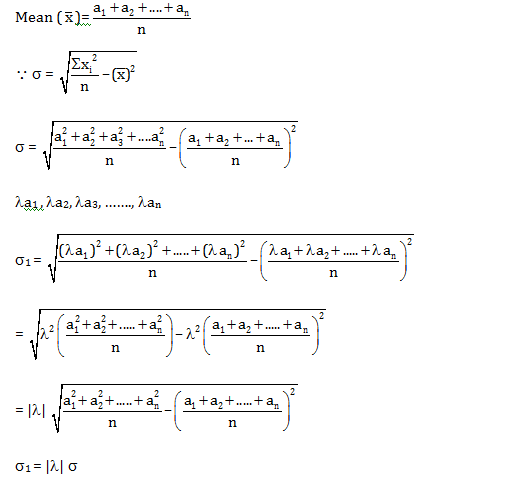Hence option (c) is correct.

Question 51. The locus of the midpoints of chords of the circle x2+y2 =1 which subtends a right angle at the origin is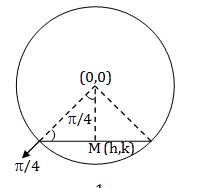1. a. x2+y2 = (1/4)
2. b. x2+y2 = (1/2)
3. c. xy = 0
4. d. x2 – y2 = 0

Solution:

$$OM=\sqrt{(0-h)^{2}+(0-k)^{2}}$$

$$OM=\sqrt{(h)^{2}+(k)^{2}}$$

Therefore, In ΔOPM

$$sin\left [ \frac{\pi }{4} \right ]=\frac{Perpendicular}{hypotenuse}=\frac{\sqrt{h^{2}+k^{2}}}{1}$$

$$\Rightarrow \frac{1}{\sqrt{2}}=\frac{\sqrt{h^{2}+k^{2}}}{1}$$

Squaring both side, we get

⇒2(h2 + k2) = 1

(h, k)

$$\rightarrow$$
(x, y)

2(x2+y2) = 1

⇒x2+y2 = 1/2

Hence option (b) is correct.

Question 52: The locus of the midpoints of all chords of the parabola y2 = 4ax through its vertex is another parabola with directrix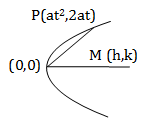1. a. x = – a
2. b. x = a
3. c. x = 0
4. d. x=-a/2

Solution:

Let midpoint of chord = (h, k)

Since h =( at2+0)/2 ⇒at2 = 2h …..(1)

K =(2at-0)/2 ⇒ at = k

⇒ t =k/a

Putting value of t in (1) we get

⇒ a(k/a)2 = 2h

⇒ k2 = 2ah ⇒ y2 = 2ax

Directrix of parabola y2 = 2ax is

x = -a/2

Hence option (d) is correct

Question 53: If [x] denotes the greatest integer less than or equal to x, then the value of the integral

$$\int_{0}^{2}x^{2}[x]dx$$
equals

1. a. 5/3
2. b. 7/3
3. c. 8/3
4. d. 4/3

Solution:

$$I=\int_{0}^{2}x^{2}[x]dx$$

$$0\leq x< 1\Rightarrow [x]=0$$

$$1\leq x< 2\Rightarrow [x]=1$$

$$I=\int_{0}^{1}x^{2}[x]dx+ \int_{1}^{2}x^{2}[x]dx$$

$$I=\int_{0}^{1}x^{2}.0dx+ \int_{1}^{2}x^{2}.1dx$$

=

$$I=0+ \int_{1}^{2}x^{2}dx$$

=

$$I=0+ \left ( \frac{x^{3}}{3} \right )^{2}_{1}$$

=

$$=\frac{2^{3}}{3}-\frac{1^{3}}{3}$$

=

$$=\frac{8}{3}-\frac{1}{3}=\frac{7}{3}$$

Question 54: The number of points at which the function f(x) = max {a – x, a + x, b}, – ∞ <x <∞, 0 < a < b cannot be differentiable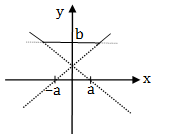1. a. 0
2. b. 1
3. c. 2
4. d. 3

Solution: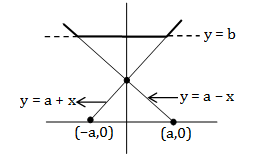f(x) ={a-x,a+x.b}

–a < x < ∞, a < a < b

y = a – x

y = a + x

y = b

Possible graph of f(x) is shown.

There are two sharp turn. Hence f(x) is not differentiable at two points.

Question 55: For non-zero vectors

$$\vec{a}$$
and
$$\vec{b}$$
if
$$\left | \vec{a}+\vec{b} \right |< \left | \vec{a}-\vec{b} \right |$$
then
$$\vec{a}$$
and
$$\vec{b}$$
are

1. a. Collinear
2. b. Perpendicular to each other
3. c. Inclined at an acute angle
4. d. Inclined at an obtuse angle

Solution:

$$\left | \vec{a}+\vec{b} \right |< \left | \vec{a}-\vec{b} \right |$$

Squaring both sides we get

$$\left | \vec{a}+\vec{b} \right |^{2}< \left | \vec{a}-\vec{b} \right |^{2}$$

$$\Rightarrow \left | \vec{a} \right |^{2}+\left | \vec{b} \right |^{2}+2\left | \vec{a} \right |.\left | \vec{b} \right |cos\alpha < \left | \vec{a} \right |^{2}+\left | \vec{b} \right |^{2}-2\left | \vec{a} \right |\left | \vec{b} \right |cos\alpha$$

(α is the angle between

$$\vec{a}$$
and
$$\vec{b}$$
)

$$\Rightarrow 2\left | \vec{a} \right |.\left | \vec{b} \right |cos\alpha < -2\left | \vec{a} \right |\left | \vec{b} \right |cos\alpha$$

$$\Rightarrow 4\left | \vec{a} \right |.\left | \vec{b} \right |cos\alpha < 0$$

$$\Rightarrow \left | \vec{a} \right |.\left | \vec{b} \right |cos\alpha < 0$$

$$\Rightarrow cos\alpha < 0$$

α is an obtuse angle.

Hence (d) option is correct.

Question 56: General solution of

$$y\frac{dy}{dx}+by^{2}=acosx,0< x< 1$$
is. Here c is an arbitrary constant

1. a.y2 = 2a(2b sinx + cosx) + ce–2bx
2. b.(4b2 + 1)y2 = 2a(sinx + 2bcosx) + ce–2bx
3. c. (4b2 + 1)y2 = 2a(sinx + 2bcosx) + ce2bx
4. d. y2 = 2a(2bsinx + cosx) + ce–2bx

Solution: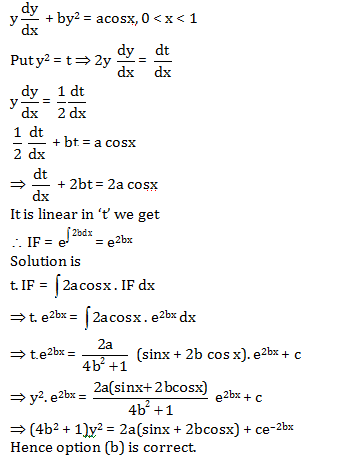Question 57: The points of the ellipse 16x2 + 9y2 = 400 at which the ordinate decreases at the same rate at which the abscissa increases is/are given by

1. a.
$$\left ( 3,\frac{16}{3} \right )\, and\, \left ( -3,\frac{-16}{3} \right )$$
2. b.
$$\left ( 3,\frac{-16}{3} \right )\, and\, \left ( -3,\frac{16}{3} \right )$$
3. c.
$$\left ( \frac{1}{16},\frac{1}{9} \right )\, and\, \left ( \frac{-1}{16},\frac{1}{9} \right )$$
4. d.
$$\left ( \frac{1}{16},\frac{1}{9} \right )\, and\, \left ( \frac{-1}{16},\frac{-1}{9} \right )$$

Solution:

Given, 16x2 + 9y2 = 400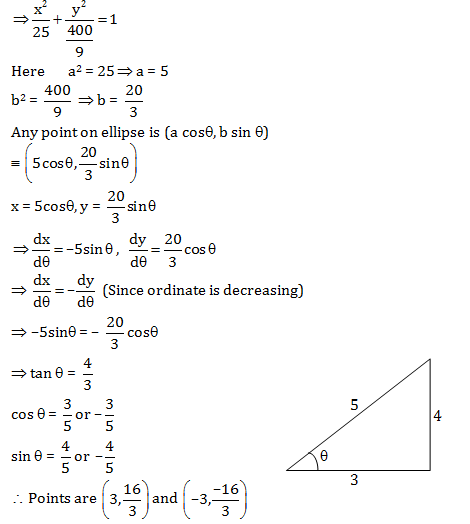Hence option (a) is correct.

Question 58: The letters of the word COCHIN are permuted and all permutation are arranged in an alphabetical order as in an English dictionary. The number of words that appear before the word COCHIN is

1. a. 96
2. b.48
3. c. 183
4. d.267

Solution:

Arranging in alphabetical order

$$\rightarrow$$
C, C, H, I, N, O

Number of words that appear before the word COCHIN is

CC ……..

$$\rightarrow$$
4!

CH ……..

$$\rightarrow$$
4!

CI ……..

$$\rightarrow$$
4!

CN ……..

$$\rightarrow$$
4!

COCHIN ……..

$$\rightarrow$$
1

Therefore, No. of words before …….. COCHIN= 4! + 4! + 4! + 4! = 96

Hence option (a) is correct.

Question 59: If the matrix

$$A=\begin{pmatrix} 2 & 0 & 0\\ 0 & 2 & 0\\ 2& 0 & 2 \end{pmatrix}$$
, then
$$A^{n}=\begin{pmatrix} a & 0 & 0\\ 0 & a & 0\\ b& 0 & a \end{pmatrix}$$
, n ∈N where

1. a. a = 2n, b= 2n
2. b. a= 2n,b= 2n
3. c.a= 2n,b=n2n-1
4. d. a=2n,b=n2n

Solution: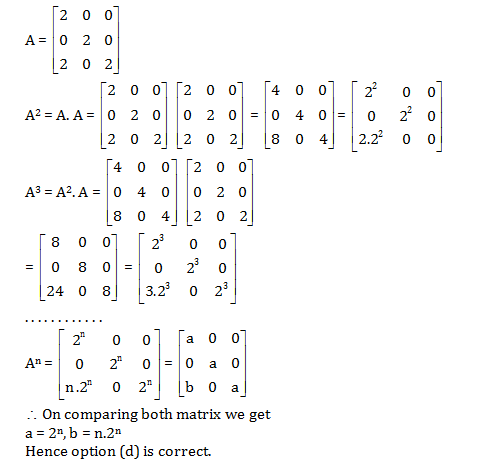Question 60: The sum of n terms of the following series; 13 +33 + 53 +73 +…. is

1. a. n2 (2n2 – 1)
2. b. n3 (n – 1)
3. c. n3 + 8n + 4
4. d. 2n4 + 3n2

Solution:

Given series is

Sn = 13 + 33 + 53 + 73 + . . . . . . . .

tr = (2r–1)3 = 8r3 – 1 – 3.2r.1(2r–1)

= 8r3–1–12r2 + 6r

Therefore,

$$S_{n}=\sum_{r=1}^{n}tr=\sum_{r=1}^{n}(8r^{3}-1-12r^{2}+6r)$$

$$=8\sum_{r=1}^{n}r^{3}-\sum_{r=1}^{n}1-12\sum_{r=1}^{n}r^{2}+6\sum_{r=1}^{n}r$$

$$=8\left [ \frac{n(n+1)}{2} \right ]^{2}-n-12\frac{n(n+1)(2n+1)}{6}+6\frac{n(n+1)}{2}$$

= 2n2(n+1)2 – n –2n(n+1) (2n+1) + 3n(n+1)

= 2n2(n2+1+2n) – n –(2n2+2n) (2n+1) + 3n2 + 3n

= 2n4 + 2n2 + 4n3 – n –4n3 – 4n2 –2n2–2n + 3n2 + 3n

= 2n4 –n2

Sn = n2(2n2–1)

Hence option (a) is correct

Question 61: If α and β are roots of ax2 + bx + c = 0 then the equation whose roots are α2 and β2 is

1. a. a2x2 – (b2 – 2ac)x + c2 = 0
2. b. a2x2 + (b2 – ac)x + c2 = 0
3. c. a2x2 + (b2 + ac)x + c2 = 0
4. d. a2x2 + (b2 + 2ac)x + c2 = 0

Solution:

α & β are roots of ax2 + bx + c = 0

Let y = x2 ⇒x = √y

Putting √y in the given equation, we get

⇒a(√y )2 + b(√y ) + c = 0

⇒ b(√y ) = –ay – c

Squaring both sides we get

⇒b2y = a2y2 + c2 +2acy

⇒a2y2 – (b2–2ac) y + c2 = 0

So the required equation is

a2x2–(b2–2ac) x + c2 = 0

Hence option (a) is correct

Question 62: If ω is an imaginary cube root of unity, then the value of (2 – ω)(2 – ω2) + 2(3 – ω)(3 – ω2) + ..... + (n – 1)(n – ω)(n – ω2) is

1. a.
$$\frac{n^{2}}{4}(n+1)^{2}-n$$
2. b.
$$\frac{n^{2}}{4}(n+1)^{2}+n$$
3. c.
$$\frac{n^{2}}{4}(n+1)^{2}$$
4. d.
$$\frac{n^{2}}{4}(n+1)-n$$

Solution: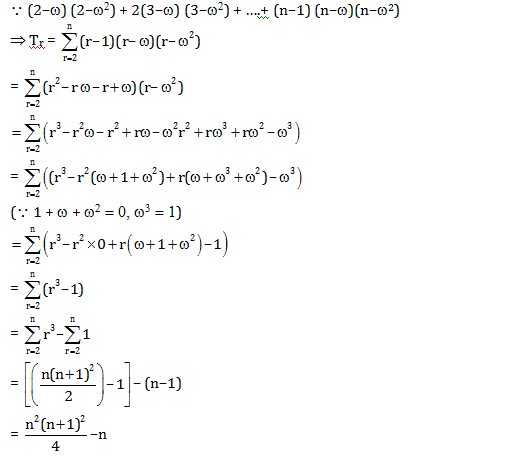Hence option (a) is correct

Question 63: If nC r–1 = 36, nCr = 84 and nCr+1 = 126 then the value of nC8 is

1. a. 10
2. b. 7
3. c. 9
4. d. 8

Solution:

Here equation (i) = 36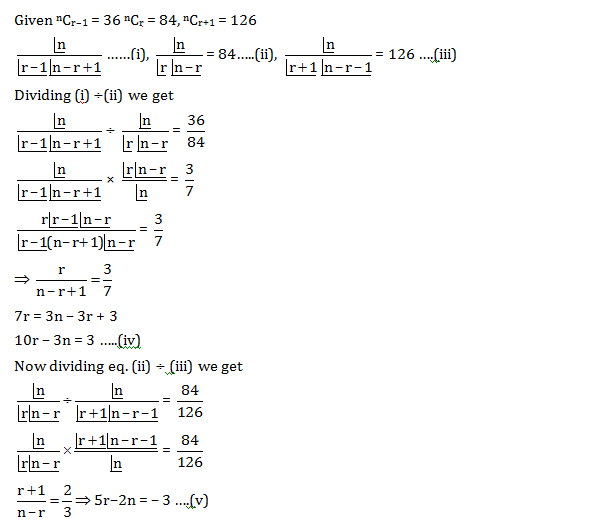Solving eq. (iv) & (v) we get r = 3 & n = 9

Therefore, nC8 = 9C8 = 9

Hence option (c) is correct.

Question 64: In a group 14 males and 6 females, 8 and 3 of the males and females respectively are aged above 40 years. The probability that a person selected at random from the group is aged above 40 years, given that the selected person is female, is

1. a. 2/7
2. b. 1/2
3. c. 1/4
4. d. 5/6

Solution:

Since there are total 14 males and 6 females in a group. In which 8 males and 3 females are aged above 40 years.

Here out of 6 females 3 are above 40 and 3 are aged below 40. So probability of person aged above 40 given female person = 1/2

Question 65: The equation x3 –yx2 + x – y = 0 represents

1. a. a hyperbola and two straight lines
2. b. a straight line
3. c. a parabola and two straight lines
4. d. a straight line and a circle

Solution:

Given equation, x3 – yx2 + x – y = 0

⇒ x2(x – y) + (x – y) = 0

⇒ (x – y) (x2 + 1) = 0

So only possibility is x – y = 0 or x2 + 1 = 0

⇒x = y or x2 + 1 = 0 (not possible)

Hence, given equation represents straight line. Hence option (B) is correct.

Question 66: If the first and the (2n+1)th terms of an AP, GP and HP are equal and their nth terms are respectively a, b, c then always

1. a. a = b = c
2. b. a
$$\geq$$
b
$$\geq$$
c
3. c. a + c = b
4. d. ac – b2 = 0

Solution:

1. Answer: (b, d)

There is mistake in question.

If there are (2n – 1)th terms instead of (2n + 1) terms then nth terms of the AP, GP and HP are the AM, GM & HM of the 1st and the last terms.

So,

$$a\geq b\geq c \: and\: ac-b^{2}(B,D)$$

Otherwise if there are (2n + 1) terms then the nth terms should be in decreasing order of AP, GP & HP.

i.e.

$$a\geq b\geq c (B)$$

Question 67: The coordinates of a point on the line x + y + 1 = 0 which is at a distance 1/5 unit from the line 3x + 4y + 2 = 0 are

1. a. (2, – 3)
2. b. (–3, 2)
3. c. (0, –1)
4. d. (–1, 0)

Solution:

1. Answer: (b, d)

Let any parametric point on the line x + y + 1 = 0 is (t, –1 –t ).

Distance of (t, –1 – t) from 3x + 4y + 2 = 0 is

$$\Rightarrow \left | \frac{3\times t\times 4(-1-t)+2}{\sqrt{3^{2}+4^{2}}} \right |=\frac{1}{5}$$

$$\Rightarrow \left | \frac{3t-4-4t+2}{5} \right |=\frac{1}{5}$$

$$\Rightarrow \left | \frac{-t-2}{5} \right |=\frac{1}{5}$$

$$\left | t+2 \right |=1$$

⇒t + 2 = 1 or t + 2 = – 1

t = – 1 or t = – 3

Therefore, Possible points on the line x + y + 1 = 0

(t, –1–t) =(–1, –1 + 1) = (–1, 0)

(t, –1–t) =(–3, –1 + 3) = (–3, 2)

Hence option (b,d) are correct

Question 68: If the parabola x2 = ay makes an intercept of length √40 unit on the line y – 2x = 1 then a is equal to

1. a. 1
2. b. – 2
3. c. –1
4. d. 2

Solution:

Parabola: x2 = ay

Line: y – 2x = 1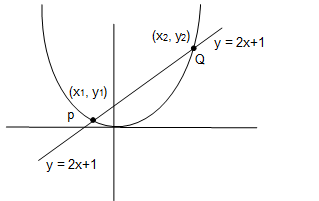Solving parabola and line

x2 = a(1 + 2x)

x2 = a + 2ax

⇒x2 – 2ax – a = 0

Let x1 and x2 are roots.

Therefore, x1 + x2 = 2a

x1 x2 = –a

Therefore,(x1 – x2)2 = (x1 + x2)2 – 4x1x2

= (2a)2 – 4(–a)

= 4a2 + 4a

(x1 – x2)2 = 4a(a + 1)

Point (x1, y1) lie on line y = 2 x + 1

Therefore, y1 = 2x1 + 1

Also point (x2, y2) line on line y = 2x + 1

y2 = 2x2 + 1

y1 – y2 = 2(x1 – x2)

(y1 – y2)2 = 4 (x1 – x2)2 = 4. 4a(a + 1)

(y1 – y2)2 = 16 a(a + 1)

Length PQ=

$$\sqrt{(x_{1}-x_{2})^{2}+(y_{1}-y_{2})^{2}}$$

=

$$\sqrt{4a(a+1)+16a(a+1)}$$

PQ=

$$\sqrt{20a(a+1)}$$

$$\Rightarrow \sqrt{40}=\sqrt{20a(a+1)}$$

Squaring both sides we get

⇒40 = 20 a(a + 1)

⇒2 = a(a + 1)

⇒a2 + a – 2 =0

⇒ (a + 2) (a – 1) =0

⇒a = –2, 1

Hence option (A, B) are correct.

Question 69: If f(x) is a function such that f’(x) = (x –1)2(4 – x), then

1. a. f(0) = 0
2. b. f(x) is increasing in (0, 3)
3. c. x = 4 is a critical point of f(x)
4. d. f(x) is decreasing in (3, 5)

Solution:

Since, f ‘(x) = (x – 1)2 (4 – x)

For critical point put f ‘(x) = 0

⇒ (x – 1)2 (4 – x) = 0

⇒ x = 1, 4

Therefore x = 1 & 4 are critical point of f(x)

Now sign scheme for f ‘(x)

Therefore, f(x) is increasing in the interval

$$(-\infty,4)$$

Hence also increasing in the interval (0, 3)

And f(x) is decreasing in the interval

$$(4,\infty)$$

Hence (B, C) option is correct.

From f ‘(x), we can’t determine f(x) uniquely so f(o) can’t be predicted.

Question70: On the ellipse 4x2 + 9y2 = 1, the points at which the tangents are parallel to the line 8x = 9y are

1. a.
$$\left ( \frac{2}{5},\frac{1}{5} \right )$$
2. b.
$$\left ( \frac{-2}{5},\frac{1}{5} \right )$$
3. c.
$$\left ( \frac{-2}{5},\frac{-1}{5} \right )$$
4. d.
$$\left ( \frac{2}{5},\frac{-1}{5} \right )$$

Solution: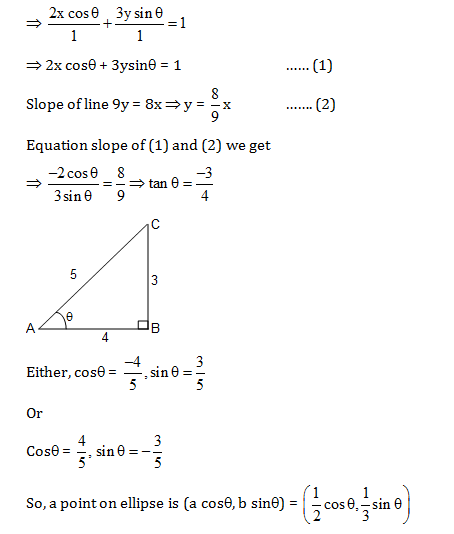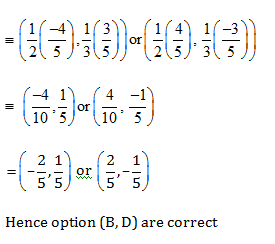Question 71: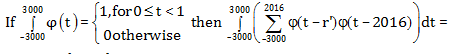1. a. a real number
2. b. 1
3. c. 0
4. d. does not exist

Solution: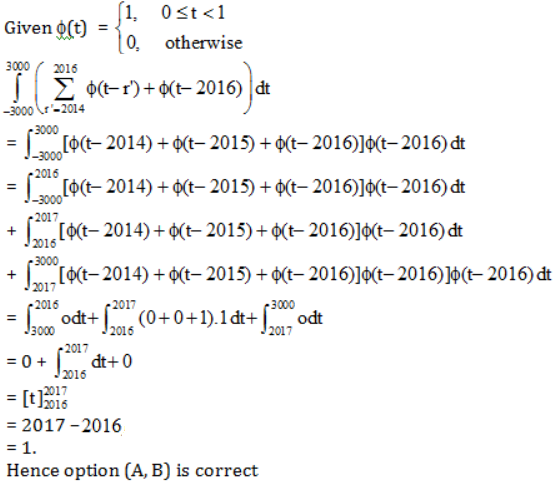Question 72: If the equation x2 + y2 –10x + 21 = 0 has real roots x = a and y = β then

1. a. 3 ≤x ≤7
2. b.3 ≤y ≤7
3. c.– 2 ≤ y ≤2
4. d.– 2 ≤ x ≤2

Solution:

Given equation is x2 + y2 – 10x + 21 = 0

⇒x2 – 10x + (y2 + 21) = 0 have roots

x = a and y = b

Therefore for real roots

⇒ (–10)2 – 4.1. (y2 + 21)≥0

⇒100 – 4y2 – 84≥0

⇒–4y2 + 16 ≥0

⇒ y2 ≤ 4

⇒-2≤y≤2

Hence option (c) is correct

Now, y2 = –x2 + 10x – 2

For real roots of y

⇒ –x2 + 10x – 21≥ 0

⇒ x2 + –10x + 21≤ 0

⇒ x2 –7x–3x + 21≤ 0

⇒ x(x–7)–3(x–7) ≤ 0

⇒ (x – 7) (x – 3) ≤ 0

⇒ 3 ≤ x ≤ 7

Option (A) is correct

Hence option (A, C) are correct

Question 73: If z = sin θ – i cos θ then for any integer n,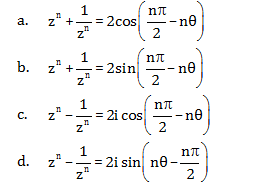Solution: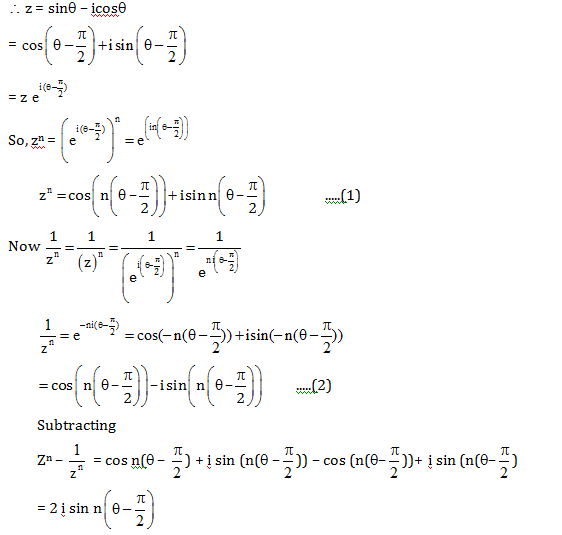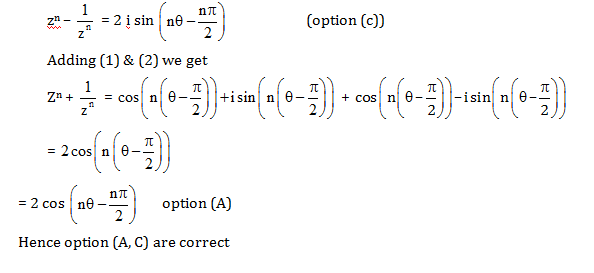Question 74: Let f : X

$$\rightarrow$$
X be such that f(f(x)) = x for all x ∈ X and X
$$\subseteq$$
R, then

1. a. f is one-to-one
2. b. f is onto
3. c. f is one-to-one but not onto
4. d. f is onto but not one-to-one

Solution:

f(f(x)) = x

$$\forall x\epsilon X \: and \: x\subseteq R$$

So, f(x) = f–1(x)

⇒f(x) is self inverse

Hence f(x) is one-one and onto

Therefore, option (A, B) is correct.

Question 75: If A, B are two events such that

$$P(A\cup B) \geq \frac{3}{4}\: and \; \frac{1}{8}\leq P(A\cap B)\leq \frac{3}{8}$$
then

1. a.
$$P(A)+P(B)\leq \frac{11}{8}$$
2. b.
$$P(A).P(B)\leq \frac{3}{8}$$
3. c.
$$P(A)+P(B)\geq \frac{3}{8}$$
4. d. None of these

Solution: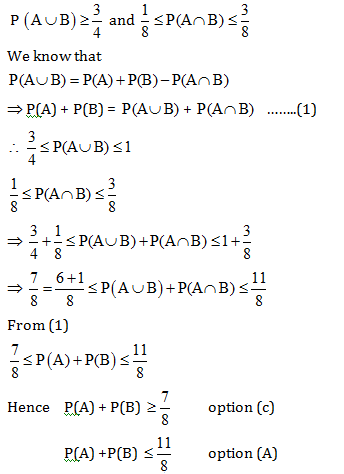### WBJEE 2016 Maths Question Paper with Solutions